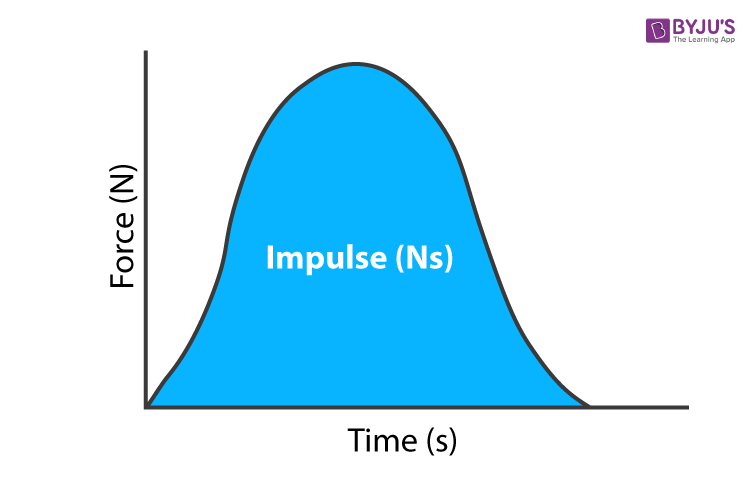Checkout JEE MAINS 2022 Question Paper Analysis : Checkout JEE MAINS 2022 Question Paper Analysis :

# What is the Unit of Impulse

The SI unit of impulse is the newton second (N⋅s). The impulse applied to an object, often in the same direction, causes an equal vector shift in its linear momentum. The SI impulse unit is the second of the Newton (N.s), and the kilogramme metre per second (kg/m/s) is the dimensionally equivalent momentum unit.

An impulse is proportional to the net force of the time span during which this force is applied to the object. Below from the equation F = ma, which comes from the second law of motion of Newton, we obtain impulse.(4)(2)